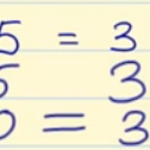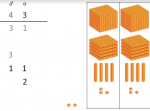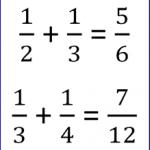Smartick is an online platform for children to master math in only 15 minutes a day

# I want to learn about: Learning Resources

Teaching resources for elementary mathematics from Smartick. Here you will find posts about teaching resources to make learning mathematics easier and more fun.

Jun09

## Properties of MultiplicationThe properties of multiplication are distributive, commutative, associative, removing a common factor and the neutral element. We are dedicating this post to examining the properties of multiplication, which are the following: Distributive property: The multiplication of a number by a sum is equal to the sum of the multiplications of this number by each one […]

Jun08

## Learn How to Subtract without Regrouping

Hello and welcome back! Today we are going to learn how to subtract without regrouping. Are you ready? Subtract Without Regrouping We’re going to follow a few steps. If you follow them well, you’ll learn how to subtract without regrouping in no time: You need to place the subtrahend below the minuend so that the ones-place […]

Jun03

## Solve and Analyze Numerical Place ValuesNumerical Place Values What are the numerical place values? Why does a number’s value change according to its position? We’ll explain everything you need to know here about understanding mathematical algorithms by working with numerical place values. In this post, we are going to learn what numerical place value is, why it’s important and how […]